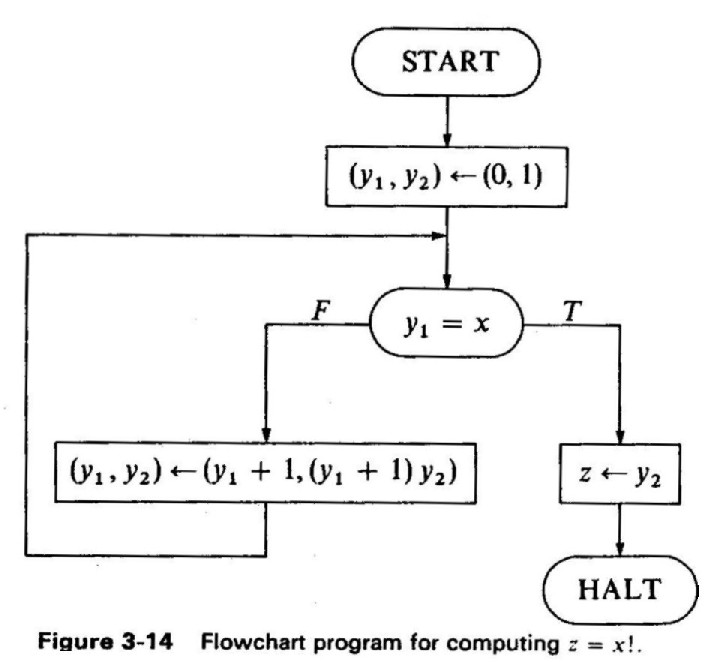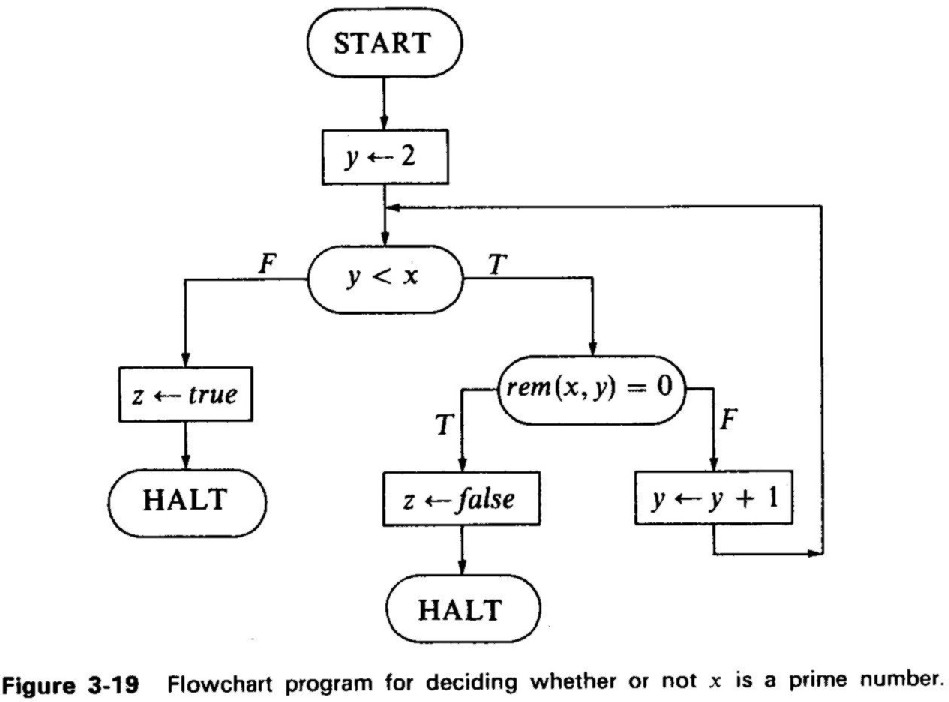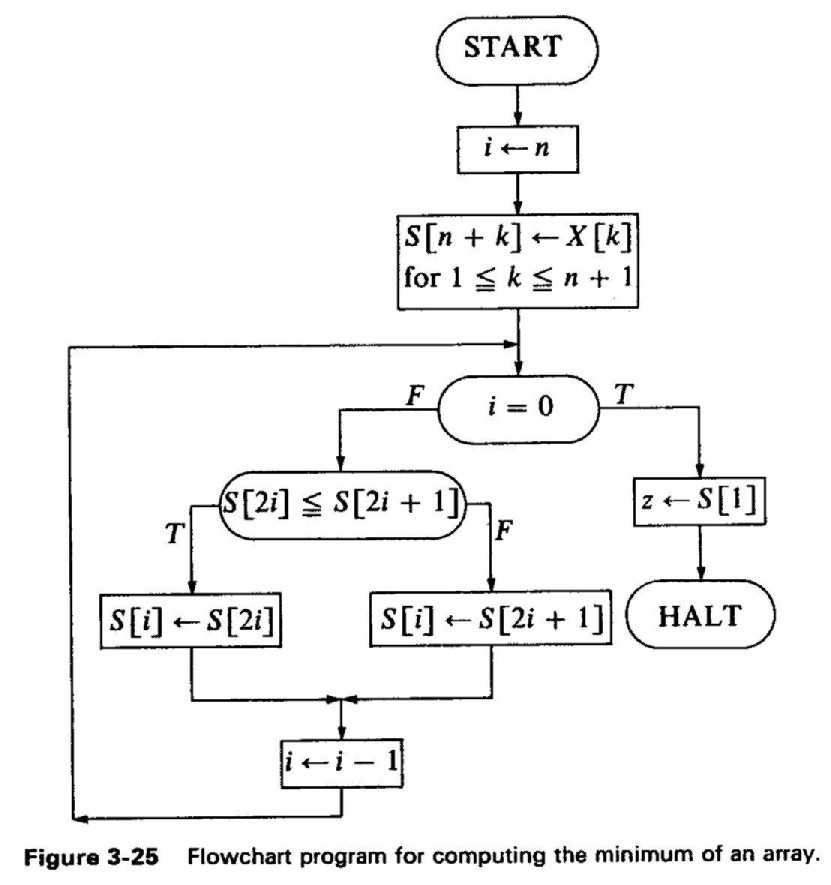# Flowcharts and Transition Graphs

## General

For each of the 3 flowcharts below you are required to:

1. Transform the flowchart into a transition graph.
2. Choose a set of cutpoints.
3. Identify the resulting set of verification paths.
4. Define the "summary guarded command" for each verification path.
5. Define an assertion (invariant) for each of the cutpoints.
6. Prove partial correctness with respect to the specification.

## I. Factorial

Specification:

• Phi(x) : x >= 0
• Psi(x,z): z = x!

All program variables are over the integers.## II. Is prime

Specification:

• Phi(x) : x >= 2
• Psi(x,z): z = true if x is prime number; z = false otherwise.

All program variables are over the integers.## III. Minimum of an array

X is a given array of real numbers: X, X, ... X[n+1],  n>=0.

Specification:

• Phi(n,X) : n >=0
• Psi(n,X,z): z = min(X, X, ... X[n+1]).## Hand-in and due date

Thursday 20/3/2003 at 11:00 (hand-in in class).